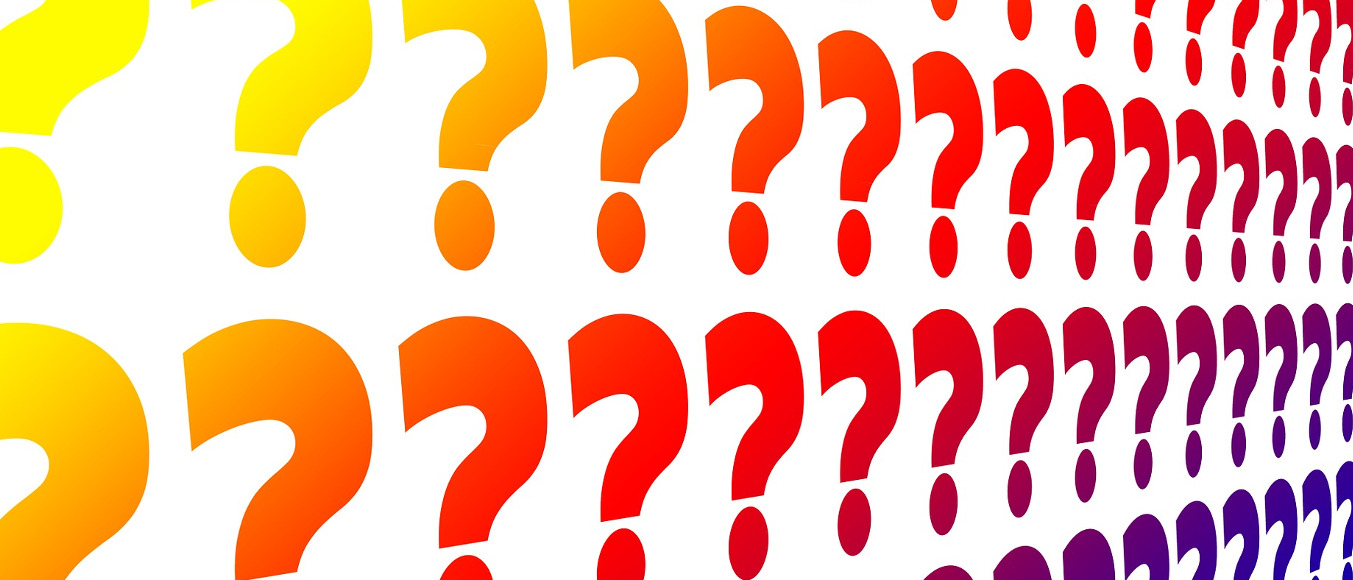# Puzzle Solutions, Issue 03

Warning: Contains SPOILERS!These solutions relate to puzzles found in Issue 03 of the magazine.

792

### Chessboard Squares

There are 64 1×1 squares, 49 2×2 squares, 36 3×3 squares, 25 4×4 squares, 16 5×5 squares, 9 6×6 squares, 4 7×7 squares and 1 8×8 square on a chessboard.
This can be shown by counting how many positions the top left corner of the square can sit on. For example, the top left corner of a 5×5 square can be in the first four rows and columns of the board (otherwise the square will go off the board) and 4×4=16.
64+49+36+25+16+9+4+1=204.